# C++ 虚继承实现原理（虚基类表指针与虚基类表）

45 篇文章 2 订阅

http://blog.csdn.net/xiejingfa/article/details/48028491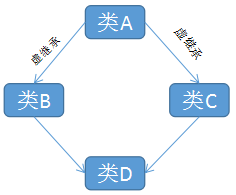1、D继承了B,C也就继承了两个虚基类指针

2、虚基类表存储的是，虚基类相对直接继承类的偏移（D并非是虚基类的直接继承类，B,C才是）

    #include<iostream>
using namespace std;

class A  //大小为4
{
public:
int a;
};
class B :virtual public A  //大小为12，变量a,b共8字节，虚基类表指针4
{
public:
int b;
};
class C :virtual public A //与B一样12
{
public:
int c;
};
class D :public B, public C //24,变量a,b,c,d共16，B的虚基类指针4，C的虚基类指针
{
public:
int d;
};

int main()
{
A a;
B b;
C c;
D d;
cout << sizeof(a) << endl;
cout << sizeof(b) << endl;
cout << sizeof(c) << endl;
cout << sizeof(d) << endl;
system("pause");
return 0;
}  

### 二： 从内存布局看C++虚继承的实现原理

1、VS2012使用命令行选项查看对象的内存布局

c1 [filename].cpp /d1reportSingleClassLayout[className]

2、查看普通多继承子类的内存布局

    /**
普通继承（没有使用虚基类）
*/

// 基类A
class A
{
public:
int dataA;
};

class B : public A
{
public:
int dataB;
};

class C : public A
{
public:
int dataC;
};

class D : public B, public C
{
public:
};  

c1  NormalInheritance.cpp /d1reportSingleClassLayoutD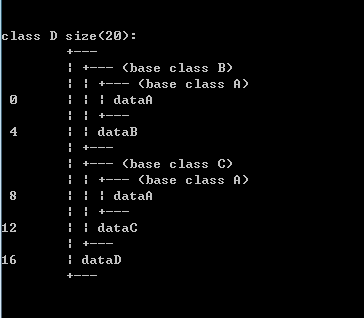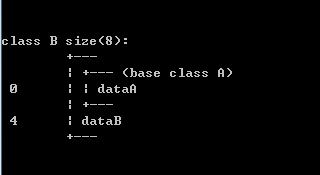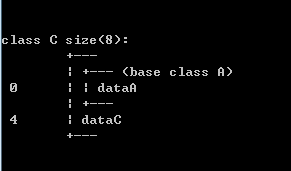/**
虚继承（虚基类）
*/

#include <iostream>

// 基类A
class A
{
public:
int dataA;
};

class B : virtual public A
{
public:
int dataB;
};

class C : virtual public A
{
public:
int dataC;
};

class D : public B, public C
{
public:
};  
VirtualInheritance.cpp和NormalInheritance.cpp的不同点在与C和C继承A时使用了virtual关键字，也就是虚继承。同样，我们看看B、C、D类的内存布局情况：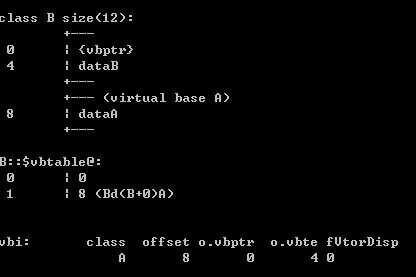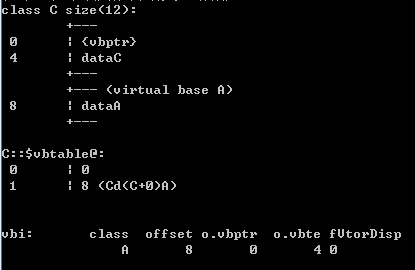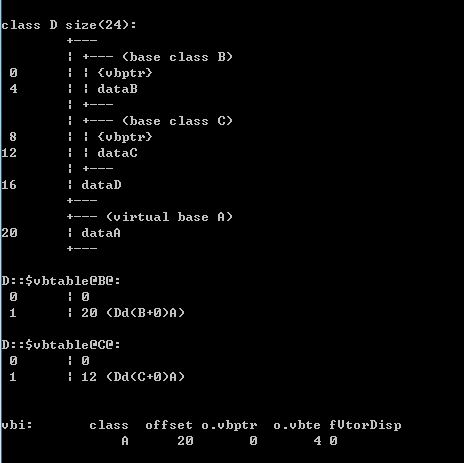vbptr：继承自父类B中的指针

int dataB：继承自父类B的成员变量

vbptr：继承自父类C的指针

int dataC：继承自父类C的成员变量

int A：继承自父类A的成员变量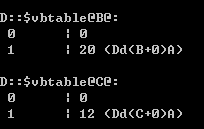int main()
{
D* d = new D;
d->dataA = 10;
d->dataB = 100;
d->dataC = 1000;

B* b = d; // 转化为基类B
C* c = d; // 转化为基类C
A* fromB = (B*) d;
A* fromC = (C*) d;

std::cout << "d address    : " << d << std::endl;
std::cout << "b address    : " << b << std::endl;
std::cout << "c address    : " << c << std::endl;
std::cout << "fromB address: " << fromB << std::endl;
std::cout << "fromC address: " << fromC << std::endl;
std::cout << std::endl;

std::cout << "vbptr address: " << (int*)d << std::endl;
std::cout << "     => " << *(int*)(*(int*)d) << std::endl;
std::cout << "     => " << *(((int*)(*(int*)d)) + 1)<< std::endl; // 偏移量20
std::cout << "dataB value  : " << *((int*)d + 1) << std::endl;
std::cout << "vbptr address: " << ((int*)d + 2) << std::endl;
std::cout << "     => " << *(int*)(*((int*)d + 2)) << std::endl;
std::cout << "     => " << *((int*)(*((int*)d + 2)) + 1) << std::endl; // 偏移量12
std::cout << "dataC value  : " << *((int*)d + 3) << std::endl;
std::cout << "dataD value  : " << *((int*)d + 4) << std::endl;
std::cout << "dataA value  : " << *((int*)d + 5) << std::endl;
}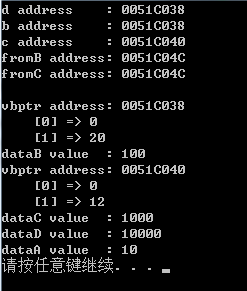12-21
08-304万+04-062096
07-19178
07-162059
03-222709
01-211284
04-231993
06-173377
10-151万+
02-11751
06-131085
04-301万+
01-151122
10-20188
12-094340
04-08661
03-311359
08-196985点击重新获取扫码支付余额充值